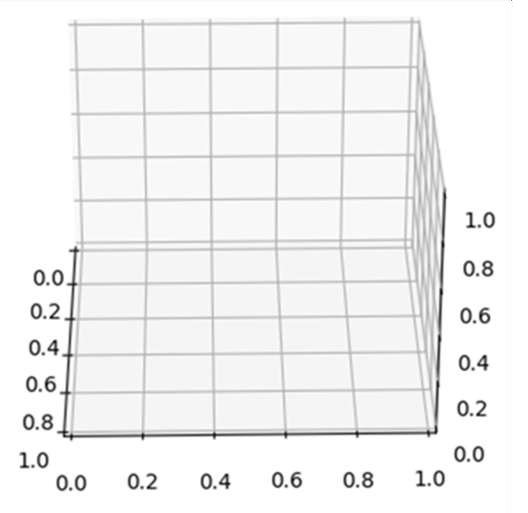# Displaying rotatable 3D plots in IPython or Jupyter Notebook

By creating a 3D projection on the axis and iterating that axis for different angles using view_init(), we can rotate the output diagram.

## Steps

• Create a new figure, or activate an existing figure.

• Add an ~.axes.Axes to the figure as part of a subplot arrangement with nrow = 1, ncols = 1, index = 1, and projection = '3d'.

• Use the method, get_test_data to return a tuple X, Y, Z with a test dataset.

• Plot a 3D wireframe with data test data x, y, and z.

• To make it rotatable, we can set the elevation and azimuth of the axes in degrees (not radians), using view_init() method.

• To show the figure, use plt.show() method.

## Example

from mpl_toolkits.mplot3d import axes3d
import matplotlib.pyplot as plt

fig = plt.figure()

X, Y, Z = axes3d.get_test_data(0.1)
ax.plot_wireframe(X, Y, Z, rstride=5, cstride=5)

# rotate the axes and update
for angle in range(0, 360):
ax.view_init(30, angle)

plt.show()

## Output